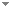Switch to: References

# Open questions about Ramsey-type statements in reverse mathematics

Bulletin of Symbolic Logic 22 (2):151-169 (2016)

 Ramsey-like theorems and moduli of computation.Ludovic Patey - 2022 - Journal of Symbolic Logic 87 (1):72-108.Ramsey’s theorem asserts that every k-coloring of $[\omega ]^n$ admits an infinite monochromatic set. Whenever $n \geq 3$, there exists a computable k-coloring of $[\omega ]^n$ whose solutions compute the halting set. On the other hand, for every computable k-coloring of $[\omega ]^2$ and every noncomputable set C, there is an infinite monochromatic set H such that $C \not \leq _T H$. The latter property is known as cone avoidance.In this article, we design a natural class of Ramsey-like theorems encompassing (...) Direct download (2 more)     Export citationBookmark1 citation The weakness of the pigeonhole principle under hyperarithmetical reductions.Benoit Monin & Ludovic Patey - 2020 - Journal of Mathematical Logic 21 (3):2150013.The infinite pigeonhole principle for 2-partitions asserts the existence, for every set A, of an infinite subset of A or of its complement. In this paper, we study the infinite pigeonhole pr... Direct download (3 more)     Export citationBookmarkThe reverse mathematics of the thin set and erdős–moser theorems.Lu Liu & Ludovic Patey - 2022 - Journal of Symbolic Logic 87 (1):313-346.The thin set theorem for n-tuples and k colors states that every k-coloring of $[\mathbb {N}]^n$ admits an infinite set of integers H such that $[H]^n$ avoids at least one color. In this paper, we study the combinatorial weakness of the thin set theorem in reverse mathematics by proving neither $\operatorname {\mathrm {\sf {TS}}}^n_k$, nor the free set theorem imply the Erdős–Moser theorem whenever k is sufficiently large. Given a problem $\mathsf {P}$, a computable instance of $\mathsf {P}$ is universal (...) Direct download (2 more)     Export citationBookmark